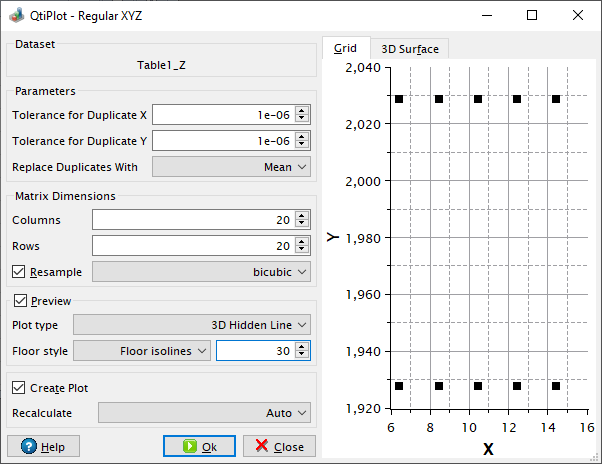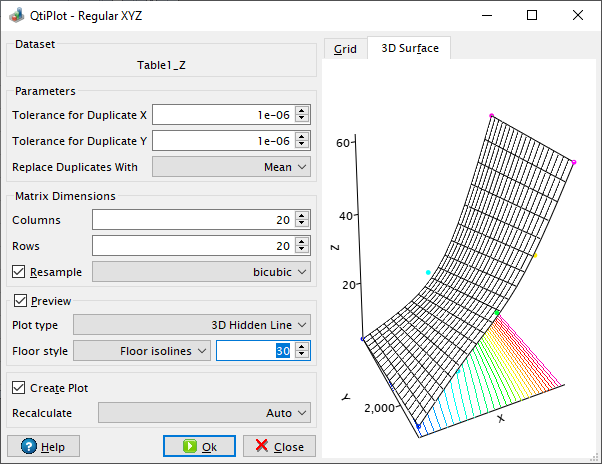# Regular XYZ Gridding Dialog

This dialog is opened when you select the Convert to Matrix -> Regular XYZ... command from the Convert to Matrix menu. It allows to customize the process of generating a new matrix from a table that contains regularly spaced XY data.

If the Preview box is checked, this dialog displays in the Grid tab a 2D scatter plot of the Y versus X data columns that are associated with the selected Z dataset. This graph allows to check the regularity of the input data: the spacing in the X and Y dimensions should be regular.

In order for the input data to be classified as regular, the values in the X and Y columns must meet specific requirements: each X value must have the same number of Y values and each Y value must have the same number of X values. In addition, both the X and the Y data values must be equally spaced: only small irregularities of the data are allowed.

Figure 5-176. The Regular XYZ Gridding dialog box: XY data grid preview.The value of the tolerance for irregularities in both X and Y directions can be customized via the Tolerance for Duplicate X and Tolerance for Duplicate Y input boxes, respectively. These two input values must be between 0 and 1.

For two adjacent data points (x1, y1, z1) and (x2, y2, z2), if |(x1 - x2)/(x1 + x2)| < ToleranceX the two X values are considered to be duplicates. The same formula is applied in the Y direction: if |(y1 - y2)/(y1 + y2)| < ToleranceY than the two Y values are also considered to be duplicates.

The Replace Duplicates With list box is useful if several Z values correspond to the same XY data pairs within the user specified tolerances. The user can choose how these Z values should be combined into a single value. The possible ways to generate a single Z value are:

Mean:

the mean of the duplicate Z values is used (default functioning mode).

Minimum Value:

the minimum Z value is used.

Maximum Value:

the maximum Z value is used.

Sum:

the sum of the duplicate Z values is used.

None:

the last of the duplicate Z values is used.

The Matrix Dimensions group box displays the number of columns and rows of the default resulting matrix. If the Resample box is checked, the Columns and Rows input boxes become editable and the user may also choose an interpolation method (either bilinear or bicubic). When the values in the Columns or Rows input boxes are modified QtiPlot automatically resizes the dimensions of the result matrix.

If the Preview box is checked, this dialog also displays a 3D plot preview of the resulting matrix together with a scatter plot of the input XYZ data points in the 3D Surface tab. The aspect of the 3D preview surface can be fully customized using the Plot type and Floor style controls.

Figure 5-177. The Regular XYZ Gridding dialog box: 3D surface preview.If the option Create Plot is checked, QtiPlot also creates a 3D plot window of the result matrix when the OK button is pressed, using the settings specified in the Plot type and Floor style controls.